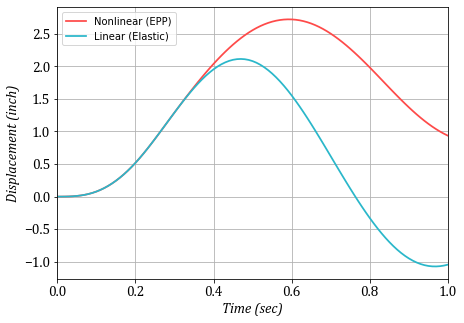# Elastoplastic SDOF system¶

#### March 2020, By Amir Hossein Namadchi¶

This is an OpenSeesPy simulation of a simple SDOF system with elastoplastic behavior mentioned in Dynamics of Structures book by Ray W. Clough and J. Penzien. This example has been solved in the book, so the result obtained here can be compared with the reference.In :
import numpy as np
import openseespy.opensees as ops
import matplotlib.pyplot as plt

In :
## Units
inch = 1               # inches
kips = 1              # KiloPounds
sec = 1               # Seconds

lb = kips*(sec**2)/inch    # mass unit (derived)


Model specifications are defined as follows:

In :
m = 0.1*lb              # Mass
k = 5.0*(kips/inch)     # Stiffness
c = 0.2*(kips*sec/inch) # Damping
dy_p = 1.2*inch         # Plastic state displacment
alpha_m = c/m           # Rayleigh damping ratio

# Variation of p(t) in tabular form
[0.1, 5],
[0.2, 8],
[0.3, 7],
[0.4, 5],
[0.5, 3],
[0.6, 2],
[0.7,1],
[0.8, 0]
])

# Dynamic Analysis Parameters
dt = 0.01
time = 1.0


### Analysis¶

Let's wrap the whole part in a function so that different material behavior could be passed to the function:

In :
def do_analysis(dt, time, material_params):
ops.wipe()
ops.model('basic','-ndm',1,'-ndf',1)
time_domain = np.arange(0, time, dt)

# Nodes
ops.node(1,0.0,0.0)
ops.node(2,0.0,0.0)

ops.uniaxialMaterial(*material_params)
ops.element('zeroLength', 1, *[1,2], '-mat', 1, '-dir', 1)
ops.mass(2, m)
ops.rayleigh(alpha_m, 0.0, 0.0, 0.0)

ops.fix(1,1)
ops.timeSeries('Path', 1,
ops.pattern('Plain', 1, 1)

# Analysis
ops.constraints('Plain')
ops.numberer('Plain')
ops.system('ProfileSPD')
ops.test('NormUnbalance', 0.0000001, 100)
ops.algorithm('ModifiedNewton')
ops.integrator('Newmark', 0.5, 0.25)
ops.analysis('Transient')

time_lst =           # list to hold time stations for plotting
response =           # response params of node 2

for i in range(len(time_domain)):
ops.analyze(1, dt)
time_lst.append(ops.getTime())
response.append(ops.nodeDisp(2,1))

print('Done with ', material_params,'!')

return {'time_list':np.array(time_lst),
'd': np.array(response)}


For comparison (similar to the book), elastic analysis is also inculded:

In :
epp = do_analysis(dt, time, ['ElasticPP', 1, k, dy_p])     # Elastic-Perfectly Plastic
els = do_analysis(dt, time, ['Elastic', 1, k])             # Elastic

Done with  ['ElasticPP', 1, 5.0, 1.2] !
Done with  ['Elastic', 1, 5.0] !


### Visualization¶

In :
plt.figure(figsize=(7,5))
plt.plot(epp['time_list'], epp['d'], color = '#fe4a49', linewidth=1.75, label = 'Nonlinear (EPP)')
plt.plot(els['time_list'], els['d'], color = '#2ab7ca', linewidth=1.75, label = 'Linear (Elastic)')
plt.ylabel('Displacement (inch)', {'fontname':'Cambria', 'fontstyle':'italic','size':14})
plt.xlabel('Time (sec)', {'fontname':'Cambria', 'fontstyle':'italic','size':14})
plt.xlim([0.0, time])
plt.legend()
plt.grid()
plt.yticks(fontname = 'Cambria', fontsize = 14)
plt.xticks(fontname = 'Cambria', fontsize = 14);### Closure¶

Results obtained here with the OpenSeesPy, totally agree with the ones in the book.

• Clough, R.W. and Penzien, J., 2003. Dynamics of structures. Berkeley. CA: Computers and Structures, Inc.